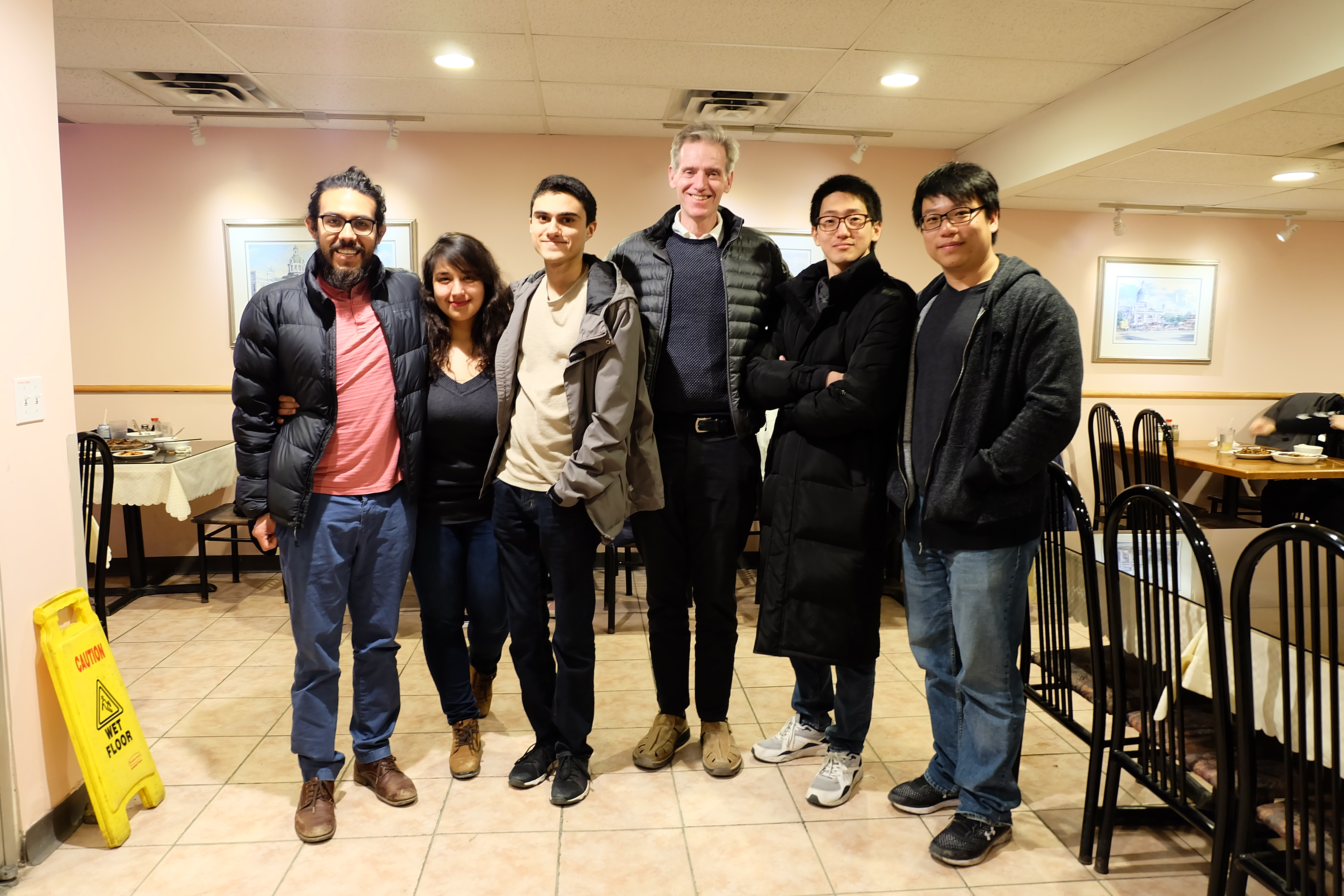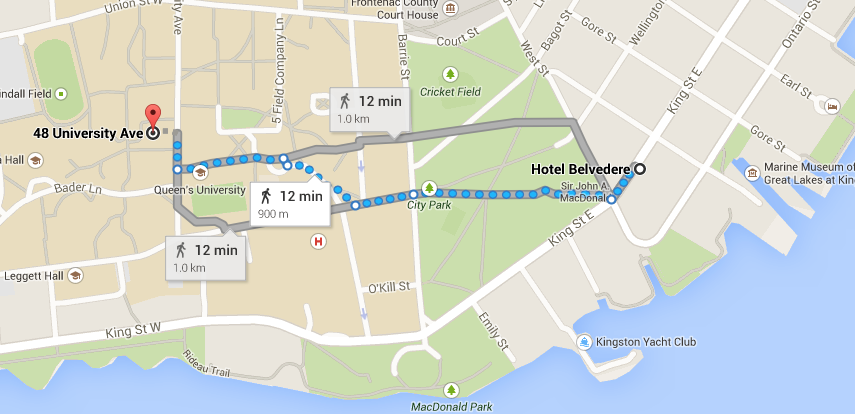# Seminar on Free Probability and Random Matrices Winter 2022

## Organizers: Jamie Mingo and Roland Speicher <!-- // // format date as dd-mmm-yy // example: 12-Jan-99 // function date_ddmmmyy(date) { var d = date.getDate(); var m = date.getMonth() + 1; var y = date.getYear(); // handle different year values // returned by IE and NS in // the year 2000. if(y >= 2000) { y -= 2000; } if(y >= 100) { y -= 100; } // could use splitString() here // but the following method is // more compatible var mmm = ( 1==m)?'Jan':( 2==m)?'Feb':(3==m)?'Mar': ( 4==m)?'Apr':( 5==m)?'May':(6==m)?'Jun': ( 7==m)?'Jul':( 8==m)?'Aug':(9==m)?'Sep': (10==m)?'Oct':(11==m)?'Nov':'Dec'; return "" + (d<10?"0"+d:d) + "-" + mmm + "-" + (y<10?"0"+y:y); } // // get last modified date of the // current document. // function date_lastmodified() { var lmd = document.lastModified; var s = "Unknown"; var d1; // check if we have a valid date // before proceeding if(0 != (d1=Date.parse(lmd))) { s = "" + date_ddmmmyy(new Date(d1)); } return s; } // // finally display the last modified date // as DD-MMM-YY // document.write( "Last modified on " + date_lastmodified() ); // -->#### Upcoming talks Previous Schedules Write to one the organizers to receive a zoom link

Thursday, April 7, 10:00 - 11:00 (Eastern), via Zoom
Monotone cumulant-moment formula and Schröder trees
The notions of cumulants in non-commutative probability can be defined combinatorially through the so-called moment-cumulant relations. These formulas write the moments of random variables as a sum of products of cumulants that is indexed by certain classes of partitions. In the cases of free and Boolean independence, Möbius inversion can be applied to obtain the inverse formulas that write cumulants in terms of moments. However, the same approach cannot be applied in the case of monotone independence. In this talk, we explain how to get the formula for the monotone case by combining two Hopf-algebraic approaches for non-commutative probability. The resulting formula is obtained in terms of Schröder trees, and it turns out to be a refinement of the formula in terms of non-crossing partitions. This is a joint work with Octavio Arizmendi (arXiv:2111.02179).

Thursday, March 31, 11:00 - 12:00 (Eastern), via Zoom (note the late start)
Benson Au (Berkeley)
Spectral asymptotics for contracted tensor ensembles
Let $\mathbf{T}_{d, N}: \Omega \to \mathbb{R}^{N^d}$ be a random real symmetric Wigner-type tensor. For unit vectors $(u_N^{(i, j)})_{i \in I, j \in [d-2]} \subset \mathbb{S}^{N-1}$, we study the contracted tensor ensemble $\left(\frac{1}{\sqrt{N}}\mathbf{T}_{d, N}\left[u_N^{(i, 1)} \otimes \cdots \otimes u_N^{(i, d-2)}\right]\right)_{i \in I}.$ For large $N$, we show that the joint spectral distribution of this ensemble is well-approximated by a semicircular family $(s_i)_{i \in I}$ whose covariance $(\mathbf{K}_{i, i'}^{(N)})_{i, i'\in I}$ is given by the rescaled overlaps of the corresponding symmetrized contractions $\mathbf{K}_{i, i'}^{(N)} = \frac{1}{d(d-1)}\langle u_N^{(i, 1)} \odot \cdots \odot u_N^{(i, d-2)}, u_N^{(i', 1)} \odot \cdots \odot u_N^{(i', d-2)}\rangle,$ which is the true covariance of the ensemble up to a $O_d(N^{-1})$ correction. In particular, if the limits $\mathbf{K}_{i, i'} = \lim_{N\to \infty} \mathbf{K}^{(N)}_{i, i'}$ exist, then the contracted tensor ensemble converges in distribution almost surely to a semicircular family $(s_i)_{i\in I}$ of covariance $(\mathbf{K}_{i, i'})_{i, i'\in I}$. In the single-matrix model $\#(I) = 1$, this implies that the empirical spectral distribution of the contracted tensor ensemble is close to the semicircle distribution $\frac{1}{2\pi \mathbf{K}_{i, i}^{(N)}} (4\mathbf{K}_{i, i}^{(N)} - x^2)_+^{1/2}\, dx$ in Kolmogorov-Smirnov distance with high probability. We further characterize the extreme cases of the variance $\mathbf{K}_{i, i}^{(N)} \in [\frac{1}{d!}, \frac{1}{d(d-1)}]$. Our analysis relies on a tensorial extension of the usual graphical calculus for moment method calculations in random matrix theory. This is joint work with Jorge Garza-Vargas.
Thursday, March 24, 10:00 - 11:00 (Eastern), via Zoom
Paul Skoufranis (York)
Non-Commutative Stochastic Processes and Bi-Free Probability
When Voiculescu introduced the notion of bi-free independence, he envisioned that the theory would model the transition between past and future states. As such, the objects under consideration were called “pairs of faces” after the Roman god of transitions Janus who is often depicted with two faces, one looking to the past and one to the future. In this talk based on on-going work, we will discuss the role bi-free probability can play in understanding the transitions in non-commutative stochastic processes.

Thursday, March 17, 10:00 - 11:00 (Eastern), via Zoom
Moritz Weber (Saarbrücken)
Quantum Cuntz Krieger Algebras
In 2020, Brannan, Eifler, Voigt and myself defined and studied quantum Cuntz-Krieger algebras. These objects are given by associating a C*-algebra to a quantum graph generalizing Cuntz-Krieger algebras.

In my talk, I will briefly recall the definitions of Cuntz-Krieger algebras and of graph C*-algebras, I will give an introduction to quantum graphs, and I will present our work on quantum Cuntz-Krieger algebras from arXiv:2009.09466. I will also mention quantum symmetries in this context: quantum automorphism groups of graphs resp. of quantum graphs are exactly the right quantum symmetries of the associated (quantum) Cuntz-Krieger algebras.

Thursday, March 3, 10:00 - 11:00 (Eastern), via Zoom
Georgios Katsimpas (York)
Bi-Free Entropy with Respect to Completely Positive Maps
The theory of free entropy developed by Voiculescu in the 1990's found several applications in the world of von Neumann algebras and remains one of the most active research areas in the theory of free probability. The non-microstate approach to free entropy, based on systems of conjugate variables and the notion of free Fisher information, was generalised by Shlyakhtenko by incorporating completely positive maps into the conjugate variable expressions. In this talk, we will develop a notion of bi-free entropy with respect to completely positive maps and discuss its applications in the operator-valued bi-free setting. This is joint work with Paul Skoufranis.

Thursday, February 17, 10:00 - 11:00 (Eastern), via Zoom
Daniel Perales (Waterloo)
On the anti-commutator of two free random variables
Let $a$, $b$ be freely independent random variables in a non-commutative probability space. Based on some considerations on bipartite graphs, we provide a formula to express the $n$-th free cumulant of the anticommutator $ab+ba$ as a sum indexed by subset $Y_{2n}$ of non-crossing partitions of $\{1,2,...,2n\}$. Specifically, $Y_{2n}$ is the set of partitions that separate odd elements and where blocks with only even elements have even size. We will study the sets $Y_{2n}$ and obtain new results regarding the distribution of $ab+ba$. For instance, the size $|Y_{2n}|$ is closely related to the case when $a$, $b$ have a Marchenko-Pastur (free Poisson) distribution of parameter 1. We will also provide a formula with the sum indexed by cacti graphs, and discuss a natural generalization to study quadratic forms in $k$ free random variables. The talk is based on the preprint arXiv:2101.09444.

Thursday, February 10, 10:00 - 11:00 (Eastern), via Zoom
Felix Leid (Saarbrücken)
Surfaced permutations and free probability
In a series of papers B. Collins, J. A. Mingo, P. Śniady and R. Speicher introduced the notion of “higher order freeness” together with a moment-cumulant formalism. The underlying combinatorics is described by “partitioned permutations” and their study led to a new $R$-transform fomula for the second order case. As the calculations for $n>2$ were way more involved than the first and second order case, it was an open problem to find the corresponding $R$-transform fomulas. In recent work with my co-authors G. Borot, S. Charbonnier, E. Garcia-Failde and S. Sadrin, we developed a combinatorial theory inspired by the notion “surfaced permutations” from the original series of papers from 2006, that allows us to capture higher genus contributions. The latter can be reformulated in terms of topological partition functions that use bosonic Fock space machinery and find $R$-transform formulas for $n>2$ . Finally we can extend the notion of higher order freeness to a higher genus version and link it to infinitesimal freeness. In my talk I will give an overview on our results with focus on the combinatorial part and free probability.

Thursday, February 3, 10:00 - 11:00 (Eastern), via Zoom
Jamie Mingo (Queen's)
Second Order Infinitesimal Freeness
Higher order infinitesimal freeness was introduced by Février in 2012. Building on the examples of Shlyakhtenko (2018) and Collins, Hasebe, and Sakuma (2018), I will present some criteria for asymptotic infinitesimal freeness of second order. I will start with the basic properties of infinitesimal freeness using the approach of upper triangular algebras. This is joint work with Pei-Lun Tseng.

## Previous Schedules

 Fall 2017 Fall 2018 Fall 2019 Fall 2021 Winter 2018 Winter 2019 Winter 2020 Winter 2021 Winter 2022 Fall 2010 Fall 2011 Fall 2012 Fall 2013 Fall 2014 Fall 2015 Fall 2016 Winter 2011 Winter 2012 Winter 2013 Winter 2014 Winter 2015 Winter 2016 Winter 2017 Fall 2003 Fall 2004 Fall 2005 Fall 2006 Fall 2007 Fall 2008 Fall 2009 Winter 2004 Winter 2005 Winter 2006 Winter 2007 Winter 2008 Winter 2009 Winter 2010

Getting to Jeffery Hall from the Hotel Belvedere